## Laboratory

Home > Laboratory > spICP-MS

### Summary of Analytical Technique

Single particle Inductively Coupled Plasma Mass Spectrometry (sp-ICP-MS) is a special type of Inductively Coupled Plasma Mass Spectrometry (ICP-MS) technique developed for the characterization (diameter, composition, etc.) of individual nanoparticles (NPs) in suspension. By flowing a solution containing single NPs or agglomerates at an appropriate concentration (about 106 particles/mL) into an inductively coupled plasma (6000-10000 K), each NP produces an ion cloud that causes a pulse of the mass signal related to the diameter of a NP. sp-ICP-MS overcome the limitations of electron microscopy requiring dry samples, by directly analyzing NPs in liquid suspensions, providing composition, mass, and diameter information for thousands of NPs per minutes. Moreover, by directly measuring the mass of each NP, sp-ICP-MS minimizes environmental parameters (e.g., temperature and vibration) often observed in other NP characterization methods based on the Brownian motion of NPs, such as NTA and DLS.

### Principle of Analytical Technique

sp-ICP-MS is based on conventional ICP-MS which consists of ionization process, sample introduction system, mass spectrometer and detector. The basic principles of ICP-MS are as follows:

#### Ionization process

The Inductively Coupled Plasma (ICP) is a module for ionization by making high temperature of plasma. The plasma is generated by combining Ar gas flowing through a series of concentric quartz tubes (the ICP torch) and an induction coil whose energy is supplied by a radio-frequency (RF) generator. Specifically, flowing argon gas is initially ionized by sparks from a Tesla coil. The resulting argon ions and electrons interact with a fluctuating magnetic field generated by the induction coil by the RF generator that radiates 0.5 to 2 kW of power at 27.12 MHz or 40.68 MHz . This interaction causes argon ions and electrons to flow in circular paths within the coil shown in Figure 1B. The resistance of argon ions and electrons to this charge flow causes ohmic heating of the plasma having a temperature of about 6000K. Since the plasma temperature is very high, it is necessary to insulate the outer quartz cylinder. Thus, thermal isolation of the outer quartz cylinder is achieved by flowing argon gas tangentially around the wall of the tube as indicated by the arrows in Figure 1A.

#### Sample introduction system

When a liquid sample enters the instrument, through a peristaltic pump, the aerosol of find droplets are generated by a meinhard nebulizer (see Figure 2). During the introduction into the plasma, the liquid droplets, containing the analyte sample is dried to a solid and then heated with gas to form atoms in the analyte. During passing through the plasma, the atoms of the analyte absorb more energy and eventually release one electron to form singly charged ions. These singly charged ions exit the plasma and enter the interface region to focus before going to the mass spectrometer system. The interface region, consisting of triple cones (sampler cone, skimmer cone, hyper skimmer cone), is the connection between the plasma region (operating atmospheric pressure) and the mass spectrometer (operating at very low pressure of 10`-8 bar).

#### Mass spectrometer and detection

Mass spectrometer can separate singly charged ions from each other by elemental mass. Commercial ICP-MS systems use three main types of mass spectrometers: quadrupole, time-of-flight, and magnetic sector. On NEXION 300D instrument, the mass spectrometer is quadrupole. Under the control of the instrument software, the mass spectrometer can move to m/z necessary to measure the elements of interest in the analyzed sample. After separation by the mass spectrometer, the selected ions hit the active surface of the detector and generate measurable electronic signals.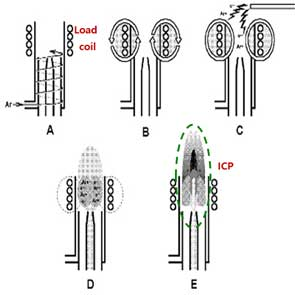Figure 1. Inductively coupled plasma generation process (Images from PerkinElmer).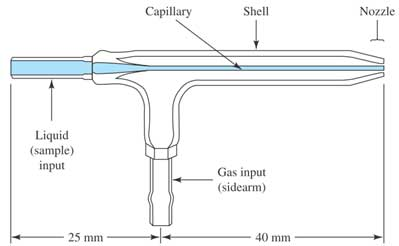Figure 2. Meinhard nebulizer. The nebulizing gas flows through an opening that concentrically surrounds the capillary. This reduces the pressure at the tip and causes aspiration of the sample. The high-velocity gas at the tip breaks up the solution into mists. (Courtesy of J. meinhard Associates, Inc.) .

#### Difference between conventional ICP-MS and sp-ICP-MS

Conventional ICP-MS is used for quantifying dissolved ions in samples. Since the ions are homogeneously distributed in the dissolved ion samples, the mass signal of the dissolved elements entering the plasma and traveling to the detector per unit time, is relatively constant. Thus, the generatein the nanoparticle solutd ions produce a continuous signal over time (Figure 3). In sp-ICP-MS, on the other hand, suspension solution of nanoparticles enters the system and the mass signal is not continuous (Figure 4). Each nanoparticle is ionized to produce an ion cloud. Each ion cloud produces a signal pulse, so the signal in the nanoparticles solution has many peaks equivalent to many nanoparticles (Figure 4).

Two important time-related terms in sp-ICP-MS are “dwell time” and “settling time”. According to Chady et al , the time it takes for a detector (e.g. quadrupole) to analyze each m/z before moving on the next m/z is called "dwell time". After each dwell time measurement, the electronics need some time to stabilize before the next measurement. This stabilization time is called “settling time" and is overhead and processing time.

Taking into account the effect of dwell time and settling time during ICP-MS measurements, some signals may be lost at settling time. In the case of dissolved ions in conventional ICP-MS, this loss is not significant, since the solution is homogeneous and the signal is continuous. For nanoparticle suspension solutions of sp-ICP-MS, this loss is significant due to the discrete signals of the nanoparticles (Figure 4). On NEXION 300D, the settling time is zero, minimizing the losses mentioned above.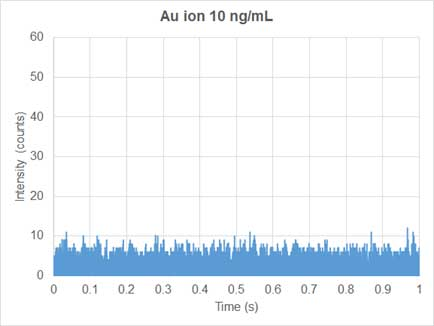Figure 3. ICP-MS 1 second data for dissolved gold ions at 10 ng/mL (Data by Tung X. Trinh).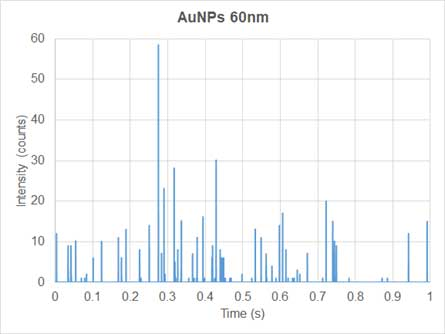Figure 4. ICP-MS 1 second data for 60 nm gold nanoparticles at 0.25 ng/mL (Data by Tung X.Trinh)

### Theory of sp-ICP-MS

sp-ICP-MS, assumes that each single particle produces a signal pulse called particle event, under three conditions(Figure 3): 1)the dwell time is short so that one or more particle event do not overlap; 2) the flow rate of nanoparticle suspension solution is constant; 3) the nanoparticle suspension solution has a low particle concentration so that multiple nanoparticles do not overlap within one dwell time period. If the assumption is correct, number concentration of nanoparticles (particles/mL) is directly related to the frequency of the pulse as shown in the following equation .

, where Np is the particle concentration (particles/mL), f(IP) is the frequency of particle events (number of pulses/min), FL is the sample flow rate (mL/min) and TE is the transport efficiency (%). The suspension solution of sample is introduced into the plasma by the meinhard nebulizer producing fine droplets. Only a small fraction (<20 %) of total number of droplets reaches the plasma. This ratio is called transport efficiency (TE). Transport efficiency is needed to calculate the particle concentration (number) of sample.

sp-ICP-MS measures the pulse signal of nanoparticles. In order to relate pulse to the diameter of nanoparticles, calibration curves of intensity - particle mass and mass-diameter equations are used. First, we need to construct a dissolved ion calibration curve that correlates the signal intensity (counts) with the mass concentration (ng/mL) of the dissolved analyte. Secondly, the mass of particles (ng/event) is related to the concentration of dissolved analyte by the thet equation below :

, where W is the particle mass (ng/event), TE is the transport efficiency (%), FL is the sample flow rate (mL/min), DT is the dwell time (min/event), and C is the mass concentration of analyte (ng/mL). Based on the calibration curve of signal intensity-dissolved mass concentration and the mass equation per particles-mass concentration, a calibration curve is constructed for signal intensity and mass per particle. Figure 5 shows an example of a corresponding calibration curve.

allows user to calculate particle mass by knowing the signal intensity using the following equation:

,where mPis the particle mass, IPis the signal intensity of particle,Ibkgd is the background intensity, IE is the ionization efficiency (%), a and b are the slope and y-intercept of intensity-mass of particle calibration curve. The ionization efficiency (IE) is 100% for silver and gold nanoparticles [8, 9, 10] with particle mass, and the diameter of particle can be calculated using the mass-diameter equation

In order to determine the transport efficiency (TE) of sp-ICP-MS, Pace and colleges suggested two methods: particle size method and particle frequency method (or particle concentration method). Both methods require a well-characterized reference nanoparticle sample.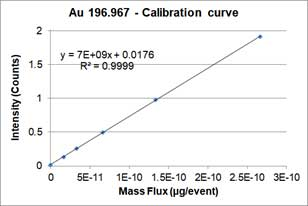Figure 5. A calibration curve that correlates the intensity and mass per event of gold nanoparticles(Data of AuNPs, dwell time is 50us, flow rate is 0.171 mL/min, date 2018.09.14, by Tung X. Trinh).

The particle size method requires two calibration curves. One is the particle calibration curve and the other is dissolved ions calibration curve. Particle calibration curve is made by measuring sp-ICP-MS of well-characterized reference nanoparticle sample (i.e., the particles are spherical and have a narrow size distribution). The equation or particle calibration curve is:

,where Y is the signal intensity (counts), W is the mass per event, b is the background noise of instrument, a_NP is the slope of calibration curve. Below is an example of a particle calibration curve measured at 60nm-AuNPs.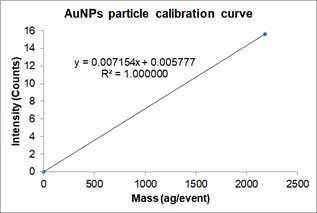Figure 6. A particle calibration curve made of 60nm-AuNPs. The unit ag is 10^(-18) g. (Data in AuNPs, dwell time is 50us, flow rate is 0.171 mL/min, date 2018.09.14, by Tung X. Trinh)

Dissolved ions calibration curves are made by measuring sp-ICP-MS of standard dissolved ion sample at different known concentrations and has a linear equation form as a particle calibration curve. Figure 7 shows an example of the dissolved calibration curve for gold ions.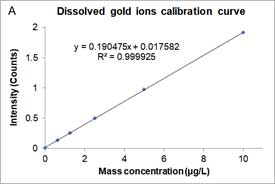Figure 7. The Original dissolved ions calibration curve of mass concentration and intensity(A) and the converted dissolved ions calibration curve of mass per event and intensity (B). (Data of gold ions standards, dwell time is 50us, flow rate is 0.171 mL/min, date 2018.09.14, by Tung X. Trinh).

Figure 7A is the mass concentration dissolved calibration curve that correlates the mass concentration (μg/L) of dissolved gold ions with the intensity of instrument. Figure 7B is the converted calibration curve in which the mass concentration (μg/L) is converted to mass per event (ag/event) by multiplying each dissolved concentration by the sample flow rate and the dwell time. Compared with Equation 2, this transformation only considers the flow rate and dwell time, since the value of transport efficiency is not yet known. Thus, assuming that transport efficiency is the same for dissolve ions and nanoparticles, and calibration curves or dissolve ions (Figure 7B) and nanoparticles (Figure 6) should be equivalent. The y-intercept difference between the two calibration curves is negligible because it is the background intensity of the instrument. When converting the mass concentration calibration curve (Figure 7A) to mass per event calibration curve (Figure 7B), the slopes of the two calibration curves are different, because we the transport efficiency is not taken into account. The ratio between the two slopes of the calibration curve is the transport efficiency :

,where TE is transport efficiency, adiss is the slope of dissolved ions calibration (mass per event vs intensity), and aNP is the slope of particle calibration curve. Transport efficiency can be calculated from the slopes of dissolved gold ions calibration curve (Figure 7B) and the particle calibration curve (Figure 6):

In the particle concentration method, measure the pulse frequency (pulse/min) where the particle concentration is equal to the number of particles/min of the known nanoparticle suspension solution. The transport efficiency can be obtained by dividing the measured particle number by the total particle number of particles introduced into sp-ICP-MS instrument.

### Limitations of sp-ICP-MS

The size limit of sp-ICP-MS is 10 nm to 2000 nm. The lower diameter limit is affected by the background/noise of the instrument. Cornelis and colleagues demonstrated the ability to measure 10 nm gold nanoparticles by deconvolution method to separate noise and signals from small nanoparticles . The upper diameter limit is affected by the transport process. Previous studies [12, 13] showed that particles larger than 2um were removed from the spray chamber and could not reach the plasma. Therefore, the upper diameter limit of sp-ICP-MS for particles would be 2000 nm.

In addition, the mass spectrometer is used for the same measurement as the conventional ICP-MS, the sensitivity of the detector and the interference in the measurement depend greatly on the element to be analyzed.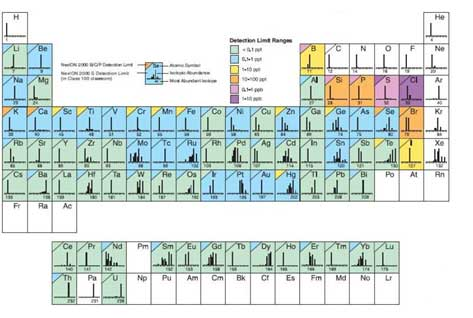Figure 8. Limit of detection depending on the measured elements. (Based on PerkinElmer's NexION 2000).

As shown in Figure 8, the detection limit varies with ionization efficiency and other factors. Analytes are separated by mass-to-charge, ratios, so interference occurs when the mass-to-charge ratio of an isobar or oxide is equal to the target element. For example, K and Ca are the same as Ar, Fe is ArO and Si, P, and S the are co-mass to charge ratios for CO, which causes large interference. Selecting isotopes avoids interference, but if choosing isotopes with low abundance can cause various problems, such as difficulty in obtaining a calibration curve. Therefore, in order to analyze the element with high interference, KED mode (Kinetic Energy Discrimination, using He gas) or DRC mode (Dynamic Reaction Cell, using NH3, O2, CH4 gas) is required for analyzing other gases in parallel. There is an appropriate mode depending on the element. For example, K and Ca causing interference by Ar, are meaningless to measure using KED mode and can only be analyzed using DRC mode using ammonia gas. In the case of sp-ICP-MS that deals with nanoparticles, when the target atom is small atomic mass, the number of particles increases with weight concentration (ppm, ppb, ppt). Therefore, the experimental conditions should be take into account, depending on the detection limit and the sensitivity of the detector.